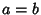## Right Hyperbola

A Hyperbola for which the Asymptotes are Perpendicular. This occurs when the Semimajor and Semiminor Axes are equal. Takingin the equation of a Hyperbola with Semimajor Axis parallel to the x-Axis and Semiminor Axis parallel to the y-Axis (i.e., vertical Directrix),therefore givesA special type of right hyperbola is the so-called Rectangular Hyperbola, which has equation.